Wednesday 25th November 2020
 CBSE Guess > Papers > Question Papers > Class XII > 2005 > Physics > Delhi Set-I PHYSICS 2005 (Set I—Delhi)
Q. 1. An electric dipole of dipole moment 20, X 10-6 C.m is enclosed by a closed surface. What is the net flux coming out of the surface? 1 Q. 2. An electron beam projected along +X-axis, experiences a force due to a magnetic field along the +Y-axis. What is the direction of the magnetic field? 1 Q. 3. The power factor of an a.c. circuit is 0.5. What will be the phase difference between voltage and current in this circuit? 1 Q. 4. Electrons are emitted from a photosensitive surface when it is illuminated by green light but electron emission does not take place by yellow light. Will the electrons be emitted when the surface is illuminated by (i) red light, and (ii) blue light. 1 Q. 5. What should be the length of the dipole antenna for a carrier wave of Frequ- ency 3 X 1O8 Hz? 1 Q. 6. Define 'electric line of force' and give its two important properties. 2 Q. 7. (a) Why does the electric field inside a dielectric decrease when it is placed in an external electric field? (b) A parallel plate capacitor with air between the plates has a capacitance of 8 pF. What will be the capacitance if the distance between the plates he reduced by half and the space between them is filled with a substance of dielectric constant K=6? 2 Or There point charges of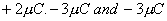are kept at the vertices, A, B and C respectively of an equilateral triangle of side 20 cm as shown in the figure. What should be the sign and magnitude of the charge to be placed at the mid-point (M) of side BC so that the charge at A remains in equilibrium? 2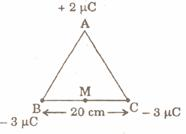Q. 8. Draw V - I graph for ohmic and non-ohmic materials. Give one example for each. 2 Q. 9. Define the terms 'Magnetic Dip' and 'Magnetic Declination' with the help of relevant diagrams. 2 Q. 10. In the figure given below, a bar magnet moving towards the right or left induces an e.m.f. in the coils (1) and (2). Find giving reason, the directions of the direction of the induced currents through the resistors AB and CD when the magnet is moving (a) towards the right, and (b) towards the left. 2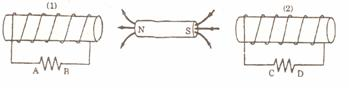Q. 11. (i) Draw the graphs showing variation of inductive reactance and Ca pacitive reactance with frequency of applied a.c. source. (ii) Can the voltage drop across the inductor or the capacitor in a series LCR circuit be greater than the applied voltage of the a.c. source? Justify youranswer. 2 Q. 12. The image of a candle is formed by a convex lens on a screen. The lower half of the lens is painted black to make it completely opaque. Draw the ray diagr- am to show the image formation. How will this image be different from the one obtained when the lens is not painted black? 2 Q. 13. An electric dipole is held in a uniform electric field. (1) Using suitable diagram, show that it does not undergo any translatory motion, and (ii) derive an expre- ssion for the torque acting on it and specify its direction. 3 Q. 14. A galvanometer with a coil of resistance 120 ohm shows full scale deflection for a current of 2.5 mA. How will you convert the galvanometer into an amm- eter of range 0 to 7.5 A? Determine the net resistance of the ammeter. When an ammeter is put in a circuit, does it read slightly less or more than the actual current in the original circuit? Justify your answer. 3 Q. 15. Define the term 'resistivity' and write its S. I . unit. Derive the expression for the resistivity of a conductor in terms of number density of free electrons and relaxation time. 3 Or State the principle of potentiometer. Draw a circuit diagram used to compare the e.m.f. of two primary cells. Write the formula used. How can the sensitivity of a potentiometer be increased? Q. 16. Explain, with the help of diagram, the principle and working of an a.c. Genera- tor. Write the expression for the e.m.f. generated in the coil in terms of its speed of rotation. 3 Q. 17. Give reasons for the following: 3(i) Long distance radio broadcasts use short-wave bands.(ii) The small ozone layer on top of the stratosphere is crucial for human survival. (iii) Satellites are used for long distance TV transmission. Q. 18. A figure divided into squares, each of size 1 mm2 is being viewed at a dista- nce of 9cm through a magnifying lens of local length 10cm, held close to the eye. (i) Draw a ray diagram showing the formation of the image. (ii) What is the magnification produced by the lens? How much is the area of each square in the virtual image? (iii) What is the angular magnification of the lens? 3 Q. 19. Ultraviolet light of wavelength 2271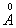from a 100 W mercury source radiates a photo cell made of molybdenum metal. If the stopping potential is 1.3 V, estimate the work function of the metal. How would the photo cell respond to high intensity (10 5 Wm -2 ) red light of wavelength 6328produced by a He - Ne laser? Plot a graph showing the variation of photoelectric current with ano- de potential for two light beams of same wavelength but different intensity. 3 Q. 20. (a) Draw a graph showing the variation of potential energy of a p of nucleons as a function of their separation. Indicate the regions in which nuclear force is (i) attractive, and (ii) repulsive. (b) Write two characteristic features of nuclear force which distinguish it from the coulomb force. 3 Q. 21. (a) Show that the decay rate 'R' of a sample of a radionuclide is related to the number of radioactive nuclei 'N' at the same instant by the expression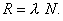(b) The half-life of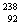U against a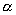- decay is 1.5 x 1017 s. What is the acti- vity of a sample of U having 25 x 1020 atoms? 3 Q. 22. Explain, with the help of a circuit diagram, how the thickness of depletion layer in a p-n junction diode changes when it is forward biased. In the follow- ing circuits which one of the two diodes is forward biased and which is rever- se biased? 3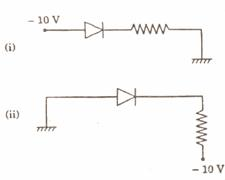Q. 23. Distinguish between, analog and digital communication. Write any two modulation techniques employed for the digital data. Describe briefly one of the techniques used. 3 Q. 24. Draw a schematic diagram of a single optical fibre structure. Explain briefly how an optical fibre is fabricated. Describe in brief, the mechanism of propag- ation of light signal through an optical fibre. 3 Q. 25. (a) With the help of a labelled diagram, explain the principle and working of a moving coil galvanometer. (b) Two parallel coaxial circular coils of equal radius 'R' and equal number of turns 'N', carry equal currents 'I' in the same direction and are separated by a distance '2R'. Find the magnitude and direction of the net magnetic field prod- uced at the mid-point of the line joining their centres. 5 Or (a) State Biot-Savart's law. Using this law, derive the expression for the magnetic field due to a current carrying circular loop of radius 'R', at a point which is at a distance 'x' from its centre along the axis of the loop. (b) Two small identical circular loops, marked (1) and (2), carrying equal currents, are placed with the geometrical axes perpendicular of each other as shown in the figure. Find the magnitude and direction of the net magnetic field produced at the point O.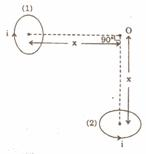Q. 26. (a) How is a wavefront different from a ray? Draw the geometrical, shape of the wavefronts when (i) light diverges from a point source, and (ii) light emerges out of a convex lens when a point source is placed as its focus. (b) State Huygens' Principle. With the help of a suitable diagram, prove Snell's law of refraction using Huygens' Principle. 5 Or (a) In Young's double slit experiment, deduce the conditions for (i) comstru- ctive, and (ii) destructive interference at a point on the screen. Draw a graph showing variation of the resultant intensity in the interference pattern against position 'x' on the screen. (b) Compare and contrast the pattern which is seen with two coherently illuminated narrow slits in Young's experiment with that seen for a coherently illuminated single slit producing diffraction. Q. 27. (a) Distinguish between metals, insulators and semiconductors on the basis of their energy bands. (b) Why are photodiodes used preferably in reverse bias condition? A photodiode is fabricated from a semiconductor with band gap of 2.8 eV. Can it detect a wavelength of 6000 nm? Justify. 5 Or (a) Explain briefly, with the help of circuit diagram, how V - I characteristics of a p-n junction diode are obtained ii (i) forward bias, and (ii) reverse bias. Draw the shape of the curves obtained. (b) A semiconductor has equal electron and hole concentration of 6 x 108 /m3 . On doping with certain impurity, electron concentration increases to 9 X1012 /m3 . (i) Identify the new semiconductor obtained after doping. (ii) Calculate the new hole concentration. Physics 2005 Question Papers Class XII Delhi Outside Delhi Compartment Delhi Compartment Outside Delhi Set I Set I Set I Set I Set II Set II Set II Set II Set III Set III Set III CBSE 2005 Question Papers Class XII